## 1. The following figure is a graph formed by stacking eight square piecespaper with the same size in turn. What should be the stacking order of thesesquare pieces of paper from top to bottom?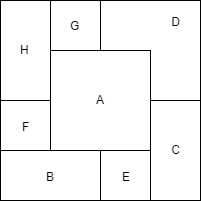• A. A-B-C-D-E-F-G-H

• B. A-D-C-H-B-E-F-G

• C. A-C-E-B-F-H-G-D

• D. A-D-G-H-F-B-E-C

C 被 E 给压住了，所以排除 C 选项

B 被 F 给压住了，所以排除 B 选项，同时排除 A 选项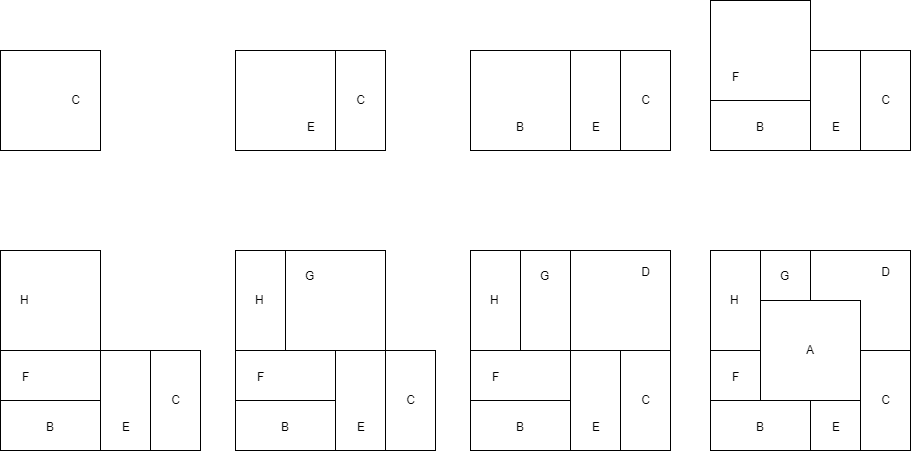## 2. According to the rule of the given figures, which figure should be the next one?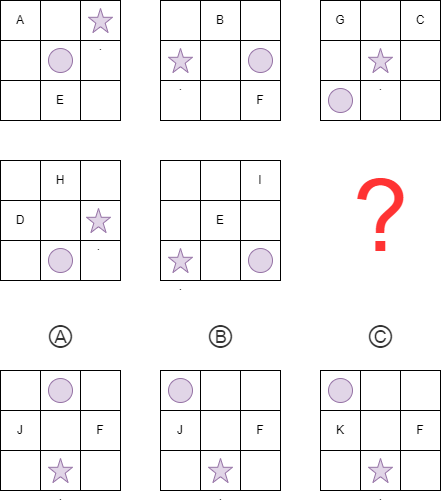• 图 1: A(r1,c1),E(r3,c2)
• 图 2: B(r1,c2),F(r3,c3)
• 图 3: C(r1,c3),G(r1,c1)
• 图 4: D(r2,c1),H(r1,c2)
• 图 5: E(r2,c2),I(r1,c3)

## 3. Below is a seven-piece puzzle, the area of the whole puzzle is 32, then,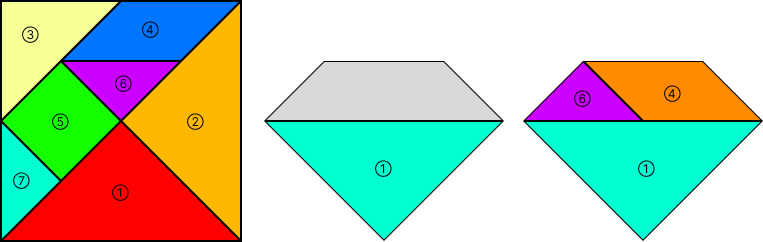1. Which integer is close to the length of the hypotenuse of ①?

2. what is the area of the parallelogram?

3. joint the above figure with some pieces in the seven-piece puzzle, and then number the pieces.

1. ① 的斜边就是正方形的边长，

2. 可以看出来 ⑥ 和 ⑦ 面积一样大，并且 ④ 的面积是 ⑥ 的 2 倍，① 的面积是 ⑥ 的 4 倍，那么整个正方形的面积： 一个三角形的面积: 平行四边形的面积:

3. 这题好像是任意发挥？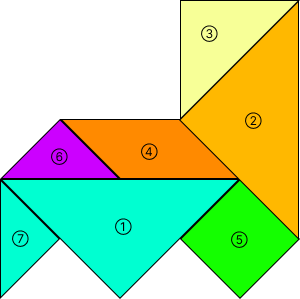## 4. Ben is Nanjinger. He has two friends -- Jack lives in Cairo and Albert live in Moscow. Moscow is 5 hours behind Nanjing and 1 hour ahead of Cairo. At 5:30 PM Moscow time,

i). what is Ben doing?

ii). what is Jack doing?

• A. Sleeping
• B. Playing football
• C. Having lunch
• D. Having dinner

1. Ben 住在南京，莫斯科比南京晚 5 小时到达 17:30，说明南京早就过了 17:30，南京此时应该是 22:30，Ben 该睡觉了。选 A

2. Jack 住在开罗，莫斯科比开罗早 1 小时到达 17:30，说明开罗还没 17:30，开罗此时应该是 16:30，吃晚饭早了点，应该在踢球吧？选 B微信